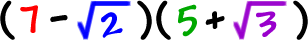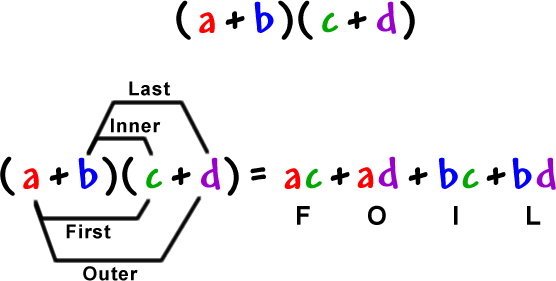We're going to need this for the next lesson.

We'll need to multiply guys likeThere's an easy process we can use called FOIL.

 F = First O = Outer I = Inner L = Last

You can use this process anytime you multiply two things that are added or subtracted inside of two sets of parentheses.  Boy, that sounds confusing!  Like this: